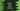# How to take inputs from user in Python## Introduction :

We have a couple of ways to take user input from the user. Using these methods, we can take input from a user in the middle or start of the program. In this post, I will show you two different ways to take user inputs in command line :

1. Using input(): input() is the easiest way to take input from a user in between program execution. We can pass one string as the argument to the input method, it can take the input and return the result to a different variable.

For example:

``````current_value = input("Enter a value : ")
print(current_value)``````

It will produce output something like below :

``````Enter a value : 20
20``````
1. Using sys.stdin : sys.stdin is used to take input from a command line directly. It actually uses input() method internally. It adds one new line after the each input it reads.

For using sys.stdin, we need to use import sys.

``````import sys

for line in sys.stdin:
if 'exit' == line.rstrip():
break
print(line)``````

In this example, we are taking the user input and printing it to the console continuously until the user prints exit

For example :

``````(base) ➜  python python3 example.py
hello
hello

world
world

!!
!!

exit
(base) ➜  python ``````

As you can see here, the program exits only when the user enters exit on the console. Else, it prints the same line that was entered.